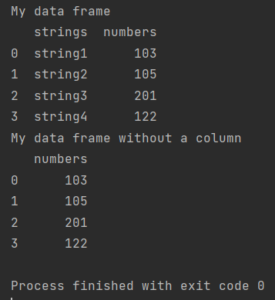Pandas How To Uncategorized 4 examples of how to delete a column

# 4 examples of how to delete a column

Here’s how to delete a column in Pandas.You already know how to add a column to the dataframe, so learn how to delete a column.

There are many ways to remove a column in Pandas. See the easiest.

## How to delete a column using del method

The easiest way is to delete a column with the del method.

```import pandas as pd

df1 = pd.DataFrame({"strings": ["string1", "string2", "string3", "string4"],
"numbers": [103, 105, 201, 122]})

print("My data frame")
print(df1)
del df1["strings"]
print("My data frame without a column")
print(df1)

How to delete a column using pop method

You can do the same using the pop method.

import pandas as pd

df1 = pd.DataFrame({"strings": ["string1", "string2", "string3", "string4"],
"numbers": [103, 105, 201, 122]})

print("My data frame")
print(df1)
df1.pop("strings")
print("My data frame without a column")
print(df1)

Remember that the syntax is a bit different than before.
Link to the description of all pop function arguments.
How to delete a column using drop method
import pandas as pd

df1 = pd.DataFrame({"strings": ["string1", "string2", "string3", "string4"],
"numbers": [103, 105, 201, 122]})

print("My data frame")
print(df1)
df1 = df1.drop(['strings'], axis=1)
print("My data frame without a column")
print(df1)

The syntax of drop is similar to the pop method. Axis = 1 removes the column. 0 drops a row.
Also check the full syntax of the drop function at this link.
How to delete a column by index
The above methods work when you know the column name. I show you how to remove a column to an index. For example, I am using the drop function to delete the first column (index 0).
import pandas as pd

df1 = pd.DataFrame({"strings": ["string1", "string2", "string3", "string4"],
"numbers": [103, 105, 201, 122]})

print("My data frame")
print(df1)
df1 = df1.drop(df1.columns[], axis=1)
print("My data frame without a column")
print(df1)

Here is a tutorial on how to delete a column in Pandas. Choose whichever method is easiest for you.
Tags: del, drop, pop

```

## How to count specific value in columnHow to count specific value in column

Here is how to count specific value in column in Pandas.

## How to check if Pandas dataframe is emptyHow to check if Pandas dataframe is empty

Here’s how to check if Pandas dataframe is empty.